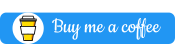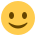#### Howdy, Stranger!

It looks like you're new here. If you want to get involved, click one of these buttons!

Supported by

# [solved] How to reset the trial counter after the practice trials

edited June 2013

In a previous discussion I received instructions in how to present to the subjects an indicator of what trial they are on and how many trials remain. However, the experiment has a practice phase and then an identical experiment phase. The instructions resulted in the correct numbers being presented in the practice phase. The indication to the subject says "That was trial number [trial_count] of [total]. There are [total - trial_count] trials to go - Where the trial count incremented from 1 to 30, and the trials remaining decremented from 29 to 0. That was fine. But then at the beginning of the experiment phase, the indication to the subject continues incrementing the trials from 31 to 60, and decrementing the trials remaining from -1 to -30.

The instructions called for a script at the beginning of the experiment with:

``````total = 30
exp.set("total", total)
``````

Than, at the beginning of the trial sequence (same for the practice loop and the experiment loop) the instructions call for a script with:

``````trial_count = self.get("count_trial_sequence")
trial_count = trial_count + 1
trials_remaining = self.get("total") - trial_count
exp.set("trial_count", trial_count)
exp.set("trials_remaining", trials_remaining)
``````

I tried changing those scripts to the following (which did not work):

For the script at the beginning of the experiment I put:

``````total = 30
trial_count = 0
exp.set("total", total)
exp.set("trial_count", trial_count)
``````

Then, for the scripts at the beginning of each trial sequence I put:

``````trial_count = trial_count + 1
trials_remaining = self.get("total") - trial_count
exp.set("trial_count", trial_count)
exp.set("trials_remaining", trials_remaining)
``````

Also, at the end of the practice loop, and before the experiment loop, I added an in-line script with this:

``````self.get("trial_count")
trial_count = 0
exp.set("trial_count", trial_count)
``````

Of course, in the experiment loop, the identical script at the beginning of the trial sequence was the same as above:

``````trial_count = trial_count + 1
trials_remaining = self.get("total") - trial_count
exp.set("trial_count", trial_count)
exp.set("trials_remaining", trials_remaining)
``````

I thought that would mean that trial_count would begin at 0, increment by 1 for the practice trial sequence, and then start back at 0 and increment by 1 for each experiment trial.

However, my changes resulted in no effect. The experiment still behaved as described in the first paragraph. Any idea what I'm doing wrong?

Thanks.

--- Bob

• edited 9:01PM

Dear Bob,

The trial_sequence item maintains its own counter, which needs to be explicitly reset. In order to do so, you could append an inline_script item to the experiment sequence, right before your experimental loop, and put the following code in its Run phase tab:

``````exp.items['trial_sequence'].count = 0
``````

The overview area should now look something like this:I hope this helps!

Best wishes,

Lotje

Did you like my answer? Feel free to• edited 9:01PM

Hi Lotje,

Yes, thanks. Your solution gave me the result I wanted. So this query is solved. I was very confused that the code referred to 'trial_sequence'. I expected it to refer to 'count_trial_sequence'. But after reading the link to more info, although I am still confused, I am less so, and I now realize I have a lot more to learn. What is a good way for someone with no programming/computer science experience or training to begin to learn more. Perhaps to actually understand programming in python?

• edited 9:01PM

Hi Bob,

I understand the confusion, it's quite tricky that simply resetting 'count_trial_sequence' to zero does not give the desired result. I think this is more a (slightly inconvenient) side-product of the way the counters are kept track of, than anything else (meaning that even experienced OpenSesame users would be confused about it). Normally, OpenSesame functions are much more intuitive; you just stumbled upon a tricky one by coincidence.

To start learning Python, we usually give the following two references:

However, for OpenSesame users it would also be very convenient if there were (a) tutorial(s) describing how to use Python inline code in combination with OpenSesame's built-in functions, something that is definitely on my to-do list!

Best,

Lotje

Did you like my answer? Feel free to